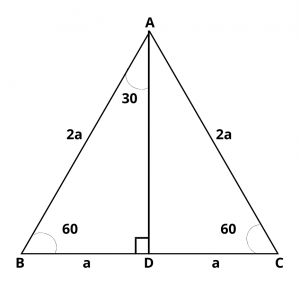# What is the Value of Cot 60 Degrees ?

## Solution :

The value of cot 60 degrees is $$1\over \sqrt{3}$$.

Proof :

Consider an equilateral triangle ABC with each side of length of 2a. Each angle of $$\Delta$$ ABC is of 60 degrees. Let AD be the perpendicular from A on BC.

$$\therefore$$   AD is the bisector of $$\angle$$ A and D is the mid-point of BC.$$\therefore$$   BD = DC = a and $$\angle$$ BAD = 30 degrees.

In $$\Delta$$ ADB, $$\angle$$ D is a right angle, AB = 2a and BD = a

By Pythagoras theorem,

$$AB^2$$ = $$AD^2$$ + $$BD^2$$  $$\implies$$  $$2a^2$$ = $$AD^2$$ + $$a^2$$

$$\implies$$  $$AD^2$$ = $$4a^2$$ – $$a^2$$ = $$3a^2$$   $$\implies$$  AD = $$\sqrt{3}a$$

Now, In triangle ADB, $$\angle$$ B = 60 degrees

By using trigonometric formulas,

$$cot 60^{\circ}$$ = $$base\over perpendicular$$ = $$b\over p$$

$$cot 60^{\circ}$$ = side adjacent to 60 degrees/side opposite to 60 degrees = $$BD\over AD$$ = $$a\over \sqrt{3}a$$ = $$1\over \sqrt{3}$$

Hence, the value of $$cot 60^{\circ}$$ = $$1\over \sqrt{3}$$